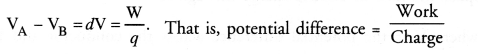# CBSE Solutions for Class 10 Physics

#### Select CBSE Solutions for class 10 Subject & Chapters Wise :

The SI unit of resistivity is ……… .

Answer :

ohm-metre (Ω m)

The resistance of a conductor depends directly on its ……… , inversely on its ……… and also on the ……… of the conductor.

Answer :

length, area of cross-section, material

According to ……… Law, the potential difference across the ends of a resistor is directly proportional to the ……… through it, provided its remains constant.

Answer :

Ohm’s, current, temperature

The SI unit of current is ……… .

Answer :

ampere

Presence of chemically inactive gases like argon and …………… prolong the life of the filament.

Answer :

Nitrogen

The rate at which electric energy is consumed or dissipated is called ……………

Answer :

Electric power

Copper and aluminium are used for electric transmission lines because both posses …………… resistivity.

Answer :

Low

Ammeter is used to measure the magnitude of …………… through any wire of a circuit.

Answer :

Electric Current

…………… measures the potential differences between two points of the circuit.

Answer :

Voltmeter

Electric current flows in the circuit from …………… terminal of cell to the …………… terminal of cell.

Answer :

Positive, Negative

Write down the relation between the potential difference between two points A and B in a conductor, work done W in moving a unit charge from point B to A and the charge q.
Or
State the relation between work, charge and potential difference for an electric circuit.
[CBSE (All India) 2009, 2013]
Or
Express work done in an electric field in terms of charge and potential difference. (CBSE 2014)

Answer :Name the instrument used to measure the electric potential difference. (CBSE 2011, 2012)

Answer :

Voltmeter.

What is meant by potential difference between two points ? (CBSE 2011)

Answer :

Work done per unit charge in moving a unit positive charge from one point to another point in an electric field is called potential difference between two points.

Is electric potential a scalar or a vector physical quantity ?

Answer :

Electric potential is a scalar physical quantity.

Define 1 volt electric potential.

Answer :

Electric potential is said to be 1 volt if 1 Joule of work is done in moving 1 coulomb charge from infinity to a point in the electric field.

What is the S.I. unit of electrical potential ? (CBSE 2005, 2012)

Answer :

volt.

State the relation between work (W), change (q) and electric potential (V).

Answer :

V = W/q.

Define electric potential.

Answer :

Electric potential at a point in an electric field is defined as the work done in moving a unit positive charge from infinity to that point in the electric field.

If the potential difference across the ends of a conductor is doubled, the current flowing through it, gets ……… .

Answer :

doubled

Mention the factor that maintains the flow of charge through a conductor. (CBSE 2013, 2014)

Answer :

Potential difference across the ends of the conductor.

Define electric current. State and define its SI unit. (CBSE 2012, 2013, 2014, 2015)

Answer :

Electric current is defined as the amount of charge flowing through a cross-section of a conductor in unit time.SI unit of electric current is ampere (A).
Electric current through a conductor is said to be 1 ampere if one coulomb charge flows through a cross-section of the conductor in one second.

Match the following columns

 Column I Column II (a) Resistance (i) Infinite (b) The rate of flow of charge (ii) Potential difference (c) The work done to move a unit charge (iii) Obstruction to the flow of current from one point to another (d) Resistance of ideal ammeter (iv) Watt (e) Resistance of ideal voltmeter (v) Electric current (f) SI unit of electric power (vi) Zero

Answer :

 Column I Column II (a) Resistance (iii) Obstruction to the flow of current from one point to another (b) The rate of flow of charge (v) Electric current (c) The work done to move a unit charge (ii) Potential difference (d) Resistance of ideal ammeter (vi) Zero (e) Resistance of ideal voltmeter (i) Infinite (f) SI unit of electric power (iv) Watt

 a. Bulb is usually filled with ampere b.  SI unit of current potential difference c.  Flow of current in a wire is due to nitrogen

Answer :

a. Bulb is usually filled with (nitrogen)
b. SI unit of current (ampere)
c. Flow of current in a wire is due to (potential difference)

### Take a Test

Choose your Test :

### Browse & Download CBSE Books For Class 10 - All Subjects

The GSEB Books for class 10 are designed as per the syllabus followed Gujarat Secondary and Higher Secondary Education Board provides key detailed, and a through solutions to all the questions relating to the GSEB textbooks.

The purpose is to provide help to the students with their homework, preparing for the examinations and personal learning. These books are very helpful for the preparation of examination.

For more details about the GSEB books for Class 10, you can access the PDF which is as in the above given links for the same.